First Steps With Deep Learning Course Deep learning

• Slides: 16First Steps With Deep Learning Course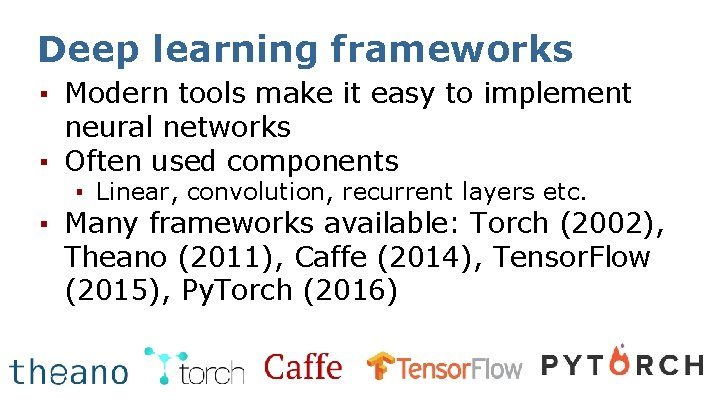Deep learning frameworks ▪ Modern tools make it easy to implement neural networks ▪ Often used components ▪ Linear, convolution, recurrent layers etc. ▪ Many frameworks available: Torch (2002), Theano (2011), Caffe (2014), Tensor. Flow (2015), Py. Torch (2016)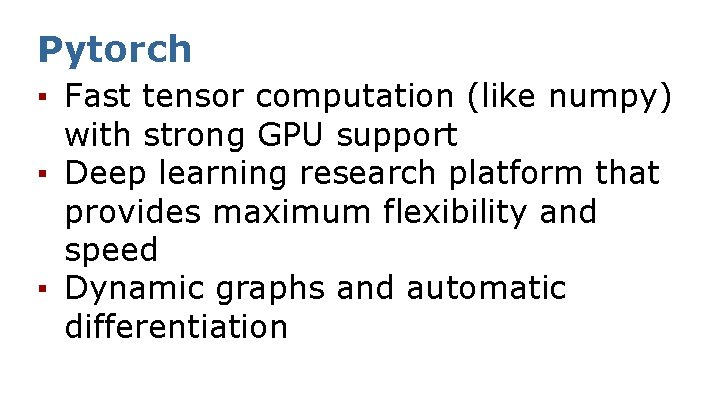Pytorch ▪ Fast tensor computation (like numpy) with strong GPU support ▪ Deep learning research platform that provides maximum flexibility and speed ▪ Dynamic graphs and automatic differentiationDevelopersOutlines ▪ Basic concepts ▪ Write a model ▪ Classification of MNIST datasetBasic Concepts torch. Tensor - similar to numpy. array autograd. Variable - wraps a Tensor and enables auto differentiation autograd. Function - operate on Variables, implements forward and backward nn. Parameter - contain Parameters and define functions on input Variables nn. Module - contain Parameters and define functions on input Variables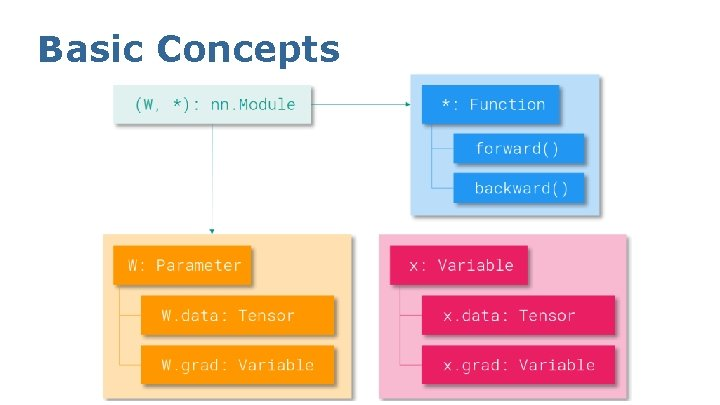Basic Concepts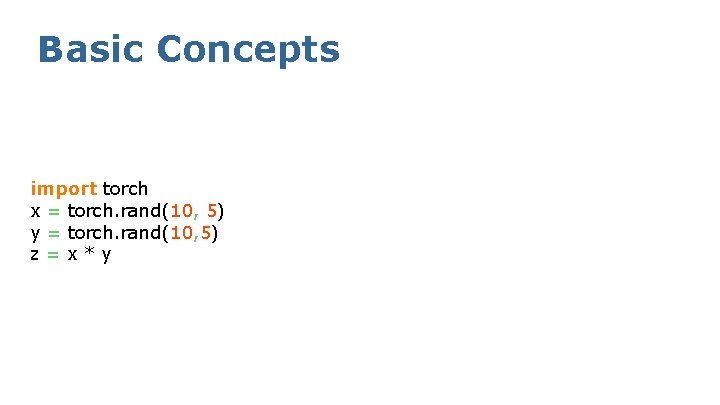Basic Concepts import torch x = torch. rand(10, 5) y = torch. rand(10, 5) z=x*y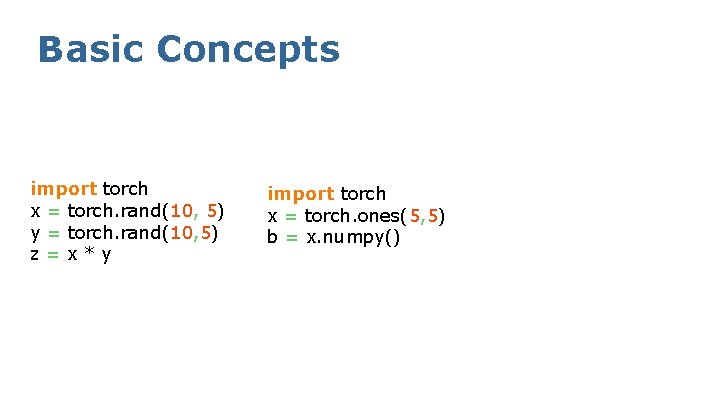Basic Concepts import torch x = torch. rand(10, 5) y = torch. rand(10, 5) z=x*y import torch x = torch. ones(5, 5) b = x. numpy()Basic Concepts import torch x = torch. rand(10, 5) y = torch. rand(10, 5) z=x*y import torch x = torch. ones(5, 5) b = x. numpy() import torch import numpy as np a = np. ones(5) x= torch. from_numpy(a)Basic Concepts import torch x= torch. rand(100, 100) x = x. cuda()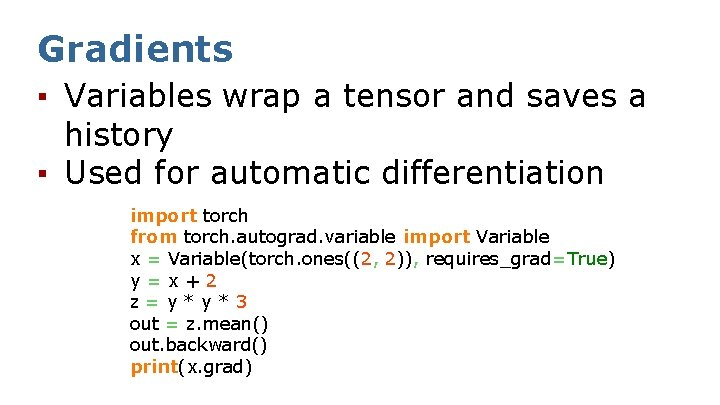Gradients ▪ Variables wrap a tensor and saves a history ▪ Used for automatic differentiation import torch from torch. autograd. variable import Variable x = Variable(torch. ones((2, 2)), requires_grad=True) y=x+2 z=y*y*3 out = z. mean() out. backward() print(x. grad)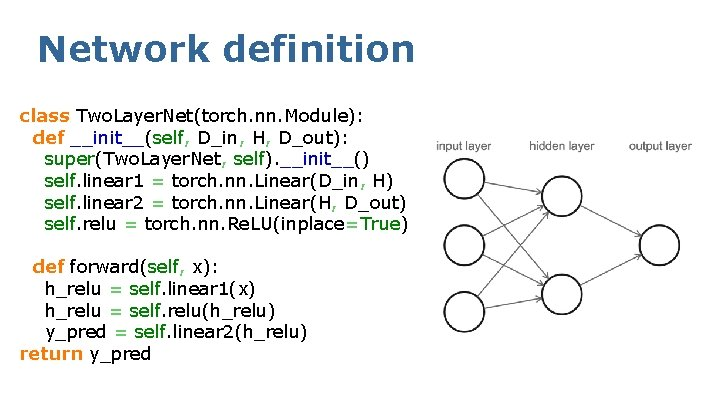Network definition class Two. Layer. Net(torch. nn. Module): def __init__(self, D_in, H, D_out): super(Two. Layer. Net, self). __init__() self. linear 1 = torch. nn. Linear(D_in, H) self. linear 2 = torch. nn. Linear(H, D_out) self. relu = torch. nn. Re. LU(inplace=True) def forward(self, x): h_relu = self. linear 1(x) h_relu = self. relu(h_relu) y_pred = self. linear 2(h_relu) return y_predOptimize the Network N, D_in, H, D_out = 64, 1000, 10 model = Two. Layer. Net(D_in, H, D_out) loss_fn = torch. nn. MSELoss(size_average=False) optimizer = torch. optim. SGD(model. parameters(), lr=1 e-4) for t in range(500): # Forward pass: Compute predicted y by passing x to the model y_pred = model(x) # Zero gradients, perform a backward pass, and update the weights. optimizer. zero_grad() loss. backward() optimizer. step()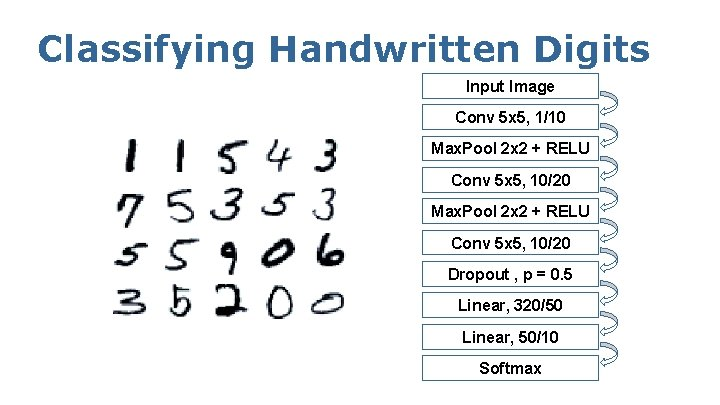Classifying Handwritten Digits Input Image Conv 5 x 5, 1/10 Max. Pool 2 x 2 + RELU Conv 5 x 5, 10/20 Dropout , p = 0. 5 Linear, 320/50 Linear, 50/10 Softmax